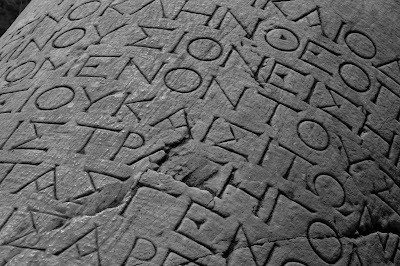7/22/2013 12:52:00 上午Photo Credit: sjkln Flickr via Compfight cc

1. Inverted iota

「℩」(inverted iota) 是希臘字母 「ι」(iota) 的倒轉版。它在語言哲學紅起來，主要得歸功於羅素（Bertrand Russell）用這個符號代表確定描述詞 “the”。羅素認為 “the F is B ” 的意思是「有一個獨一無二的 F 是 B 」，例如「那一個 Waverley 的作者是男人」（The author of Waverley is a man）即是「有一個獨一無二的 Waverley 作者是男人」。它雖然與「所有 x」(∀x) 和「有個 x」(∃x) 兩個量化詞（quantifier）類似，但兩者其實南轅北轍。譬如，在以下三行

∀x(x is presently King of France)
∃x(x is presently King of France)
℩x(x is presently King of France)

P

B(a)
(a) 是 B
[ a: the presently King of France/ Charles II"； Bx: x is Bald ]

B(℩x(x is presently King of France))
(℩x(x is presently King of France)) 是 B

[ Bx: x is Bald ]

B(℩xKx)

[ Kx: x is the presently King of France； Bx: x is Bald ]

(∃x)(Kx ∧ (∀y)(Ky→y=x) ∧ Bx)

[ Kx: x is the presently King of France； Bx: x is Bald ]

2. Lambda

λ 與 ℩ 一樣可進一步彰顯句子的內部結構。不過，它不是用來顯示的複雜結構，而是用來顯示述詞（predicate）的複雜結構。在今日最普遍的述詞邏輯系統，要翻譯「小明又肥又矮」有兩個方法：

Ha
a 是 H
[ a:小明； Hx: x 又肥又矮 ]

Fa∧Sa
a 是 F 而且 a 是S
[ a:小明； Fx: x 是肥胖的； Sx: x 是矮的 ]

(λx(Fx∧Sx))a
a 是 λx(Fx∧Sx)
[ a:小明； Fx: x 是肥胖的； Sx: x 是矮的 ]

λ 的作用範圍代表一個完整個述詞， (λx(Fx∧Sx))a 的閱讀方式其實與 Ha 很相似，只要將 H 看成複合述詞 (λx(Fx∧Sx)) 。但「(λx(Fx∧Sx))」是甚麼東西？（如果述詞指性質，）它指到某個性質：「肥並且矮」（Fx∧Sx）這個複合性質。

∼Ga

[ a:小明； Gx: x 是天才 ]

∼(λxGx)a
a 不是 λxGx
a:小明； Gx: x 是天才 ]

(λx∼Gx)a
a 是 λx∼Gx
a:小明； Gx: x 是天才 ]

(λx(∀y(Tyx→Lxy)))a
a 是這樣的：對於所有 y ，如果 y 對 a 好，則 a 喜歡 y。
[ a:小明； Tyx: y 對 x 好； Lxy: x 喜歡 y ]

$C^{2}(λx(Wx∧Vx))$
$λx(Wx∧Vx)$ 是 $C^{2}$
[ $C^{2}x$； x 是愚蠢的行為； Wx: x 犯錯； Vx: x 招惹別人]

$(Na∧K^{2}(λxNx))→(∃X)(K^{2}X∧Xa)$

[ a:小明； Nx: x 用手指挖鼻屎； $K^{2}x$: x 是不衛生的 ]

3.

∼B(℩xKx)

（It is not the case that the present King of France is bald）

(λx∼Bx)(℩xKx)

（The present King of France is such that: he is not bald）